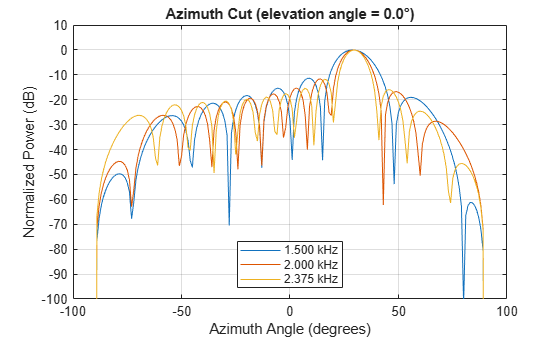## Visualization of Wideband Beamformer Performance

This example shows how to plot the response of an acoustic microphone element and an array of microphone elements to validate the performance of a beamformer. The array must maintain an acceptable array pattern throughout the bandwidth.

Note: This example runs only in R2016b or later. If you are using an earlier release, replace each call to the function with the equivalent `step` syntax. For example, replace `myObject(x)` with `step(myObject,x)`.

Create an 11-element uniform linear array (ULA) of microphones using cosine antenna elements as microphones. The `phased.CosineAntennaElement` System object™ is general enough to be used as a microphone element as well because it creates or receives a scalar field. You need to change the response frequencies to the audible range. In addition make sure the `PropagationSpeed` parameter in the array `pattern` methods are set to the speed of sound in air.

```c = 340; freq = [1000 2750]; fc = 2000; numels = 11; microphone = phased.CosineAntennaElement('FrequencyRange',freq); array = phased.ULA('NumElements',numels,... 'ElementSpacing',0.5*c/fc,'Element',microphone);```

Plot the response pattern of the microphone element over a set of frequencies.

```plotFreq = linspace(min(freq),max(freq),15); pattern(microphone,plotFreq,[-180:180],0,'CoordinateSystem','rectangular',... 'PlotStyle','waterfall','Type','powerdb')```This plot shows that the element pattern is constant over the entire bandwidth.

Plot the response pattern of an 11-element array over the same set of frequencies.

```pattern(array,plotFreq,[-180:180],0,'CoordinateSystem','rectangular',... 'PlotStyle','waterfall','Type','powerdb','PropagationSpeed',c)```This plot shows that the element pattern mainlobe decreases with frequency.

Apply a subband phase shift beamformer to the array. The direction of interest is 30° azimuth and 0° elevation. There are 8 subbands.

```direction = [30;0]; numbands = 8; beamformer = phased.SubbandPhaseShiftBeamformer('SensorArray',array,... 'Direction',direction,... 'OperatingFrequency',fc,'PropagationSpeed',c,... 'SampleRate',1e3,... 'WeightsOutputPort',true,'SubbandsOutputPort',true,... 'NumSubbands',numbands); rx = ones(numbands,numels); [y,w,centerfreqs] = beamformer(rx);```

Plot the response pattern of the array using the weights and center frequencies from the beamformer.

```pattern(array,centerfreqs.',[-180:180],0,'Weights',w,'CoordinateSystem','rectangular',... 'PlotStyle','waterfall','Type','powerdb','PropagationSpeed',c)```The above plot shows the beamformed pattern at the center frequency of each subband.

Plot the response pattern at three frequencies in two-dimensions.

```centerfreqs = fftshift(centerfreqs); w = fftshift(w,2); idx = [1,5,8]; pattern(array,centerfreqs(idx).',[-180:180],0,'Weights',w(:,idx),'CoordinateSystem','rectangular',... 'PlotStyle','overlay','Type','powerdb','PropagationSpeed',c) legend('Location','South')```This plot shows that the main beam direction remains constant while the beamwidth decreases with frequency.